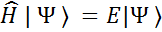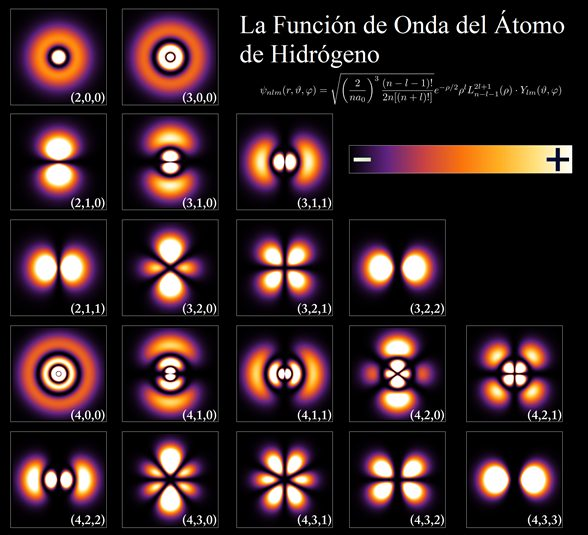HomeenLearn the concept of quantum numbers and atomic orbitals

# Learn the concept of quantum numbers and atomic orbitals

Matter is made up of tiny particles called atoms. These, in turn, are made up of a tiny positively charged nucleus, which is surrounded by a negatively charged cloud of electrons. Quantum numbers are a series of whole numbers or simple fractions that are used to describe, in a simple way, the way these electrons are structured around the nucleus . These quantum numbers allow us to define the regions in space in which electrons can be found, which are called atomic orbitals.

Understanding quantum numbers is the first step towards understanding the electronic configuration of the elements, which allows us to understand in a very simple and elegant way the transformations of matter that are studied in chemistry.

## Quantum theory and the Schrödinger equation

The physics that describes the motion of projectiles and planets stops working well when things are infinitely small. The theory that best describes matter at the atomic level is quantum theory. Just as Newton’s laws form the basis of classical physics, one of the fundamental bases of quantum theory is the Schrödinger equation, from which quantum numbers and atomic orbitals arise.

The Schrödinger equation is a differential equation that describes the behavior of electrons as waves. In its simplest version, it is written like this:Ψ is the wave function, which mathematically describes the atom.

## The wave function and atomic orbitals

Atomic orbitals arise from the Schrödinger equation or, more precisely, from the wave function. For a long time there was discussion about what the wave function meant, until it was discovered that its square, that is Ψ 2 , determines the probability of finding an electron in a certain place in space.

This allowed quantum physicists and chemists to define the regions around the nucleus where electrons are most likely to be found, hence the modern concept of an atomic orbital. In fact, an atomic orbital is defined in chemistry and quantum mechanics as the region of space in which there is a 90% probability of finding an electron .

## quantum numbers

The Schrödinger equation is not an equation that has only one solution. In fact, there are infinitely many solutions to this equation, and all of them are defined by quantum numbers. Formally, the quantum numbers arise from the different wave functions obtained by solving the Schrödinger equation for the hydrogen atom. Each combination of these numbers results in a different wave function, and therefore gives rise to a different atomic orbital.## What are the quantum numbers and how much are they worth?

There are three quantum numbers that define an atomic orbital, and an additional one that identifies a particular electron found in that orbital. These numbers are:

• Principal quantum number or energy level (n)
• Secondary quantum number or angular momentum ( l )
• Magnetic quantum number (m l )
• Spin quantum number of the electron (m s )

### Principal quantum number or energy level (n)

The principal quantum number determines, in the hydrogen atom, the energy level of an orbital. It also appears in Bohr’s atomic model and is related to the average distance of electrons from the nucleus. In atoms with more than one electron, the actual energy level of each orbital also depends on the presence of electrons in the other orbitals.

This quantum number can only take the natural numbers as values: 1, 2, 3,…

The set of orbitals that make up each main energy level is called a shell, and it is associated with a capital letter of the alphabet, starting with K.

Principal quantum number (n) one 2 3 4 5 6… Cap k L m No. EITHER P…

### Secondary quantum number or angular momentum ( l )

Angular momentum determines the shape of an orbital. Within each shell or principal energy level, there can be several different types of orbitals distinguished by the value of their angular momentum, for each of which a characteristic shape is obtained.

The possible values ​​of angular momentum depend on the principal quantum number. In fact, the angular momentum, l , can only take as a value those integers that go from zero (0) to n – 1 .

This means that at level n=1, l can only take the value of n-1=0. At level n=2, l can take 0 and 1 as values, and so on.

The angular momentum number is also called the energy subshell, and the set of orbitals within each subshell is also called the subshell. Each sublevel is also associated with a lower case letter that is related to the wave function shape. The following table shows this relationship:

Angular momentum quantum number ( l ) 0 one 2 3 4… Cap yes p d F g…

### Magnetic quantum number (m l )

The magnetic moment ml is related to the orientation in space of each orbital.

This quantum number can only take as a value those integers that are between –l and +l , including zero.

For example, if l =2 (sublevel d), m l can take the values ​​of -2, -1, 0, +1 and +2.

Each value of the magnetic moment within each subshell identifies a particular orbital. It could be said, then, that the number of possible magnetic quantum numbers indicates how many orbitals there are within each subshell.

The orientation of the orbitals is usually identified by means of the Cartesian coordinate axes, x, y and z , and this depends on the type of orbital in question.

The s orbitals are spherical, so they don’t have any preferred orientation, so there’s no need to specify their value of ml (which is 0). In the case of p orbitals, the x, y, and z directions are often assigned to the numbers -1, 0, and +1, respectively.

This is why there is a single s orbital, three p orbitals, 5 d orbitals, and so on, for each energy level (as long as n is large enough).

## n, l, and l define an orbital

From the above it follows that, to define an atomic orbital, it is only necessary to specify a particular combination of the first three quantum numbers. The following table shows some examples of the atomic orbitals of the hydrogen atom with their respective quantum numbers.

no he m l Orbital one 0 0 1s 2 0 0 2s 2 one -one 2p x 2 one 0 2p and 2 one +1 2p z 3 0 0 3s 3 one -one 3p x 3 one 0 3p x 3 one +1 3p x 3 2 -2 3d xy 3 2 -one 3d xz 3 2 0 3d and z 3 2 +1 3d x2-y2 3 2 +2 3d z2

### Spin quantum number of the electron (m s )

Finally, we have the electron spin quantum number. This quantum number indicates the direction in which each electron spins (spin means turn in English).

Electron spin can only have values ​​of +1/2 or -1/2.

The spin of an electron causes it to generate a magnetic field, and this can point in only one of two opposite directions. For this reason, spin is often represented by arrows pointing up or down, depending on whether the spin is +1/2 or -1/2.

The fact that the electron can only have 2 spin values ​​and the fact that two electrons in the same atom cannot have the same four quantum numbers (what is called the Pauli exclusion principle) means that in each orbital only There can be a maximum of two electrons with opposite spins, which are said to be paired.

## References

Atkins, Peter & Julio de Paula . (2014). Atkins’Physical Chemistry. (Rev. ed.). Oxford, United Kingdom: Oxford University Press.

Chang, R. (2008). Physical Chemistry ( 1st ed.). New York City, New York: McGraw Hill.

Epiotis, N., & Henze, D. (2003). Periodic Table (Chemistry). Encyclopedia of Physical Science and Technology , 671–695. https://doi.org/10.1016/b0-12-227410-5/00551-2

Hernandez E., D., Astudillo S., L. (2013). Knowing the quantum numbers. Chemical Education, Volume 24, Supplement 2, 485-488. Retrieved from https://www.sciencedirect.com/science/article/pii/S0187893X13725175

Pauling, L. (2021). Introduction to Quantum Mechanics: With Applications to Chemistry (First Edition). New York City, New York: McGraw-Hill.